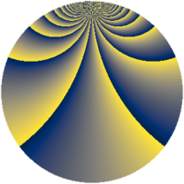# Properties

 Label 1380.2.btLevel $1380$ Weight $2$ Character orbit 1380.bt Rep. character $\chi_{1380}(127,\cdot)$ Character field $\Q(\zeta_{44})$ Dimension $2880$ Sturm bound $576$

# Related objects

## Defining parameters

 Level: $$N$$ $$=$$ $$1380 = 2^{2} \cdot 3 \cdot 5 \cdot 23$$ Weight: $$k$$ $$=$$ $$2$$ Character orbit: $$[\chi]$$ $$=$$ 1380.bt (of order $$44$$ and degree $$20$$) Character conductor: $$\operatorname{cond}(\chi)$$ $$=$$ $$460$$ Character field: $$\Q(\zeta_{44})$$ Sturm bound: $$576$$

## Dimensions

The following table gives the dimensions of various subspaces of $$M_{2}(1380, [\chi])$$.

Total New Old
Modular forms 5920 2880 3040
Cusp forms 5600 2880 2720
Eisenstein series 320 0 320

## Trace form

 $$2880q + 8q^{6} + O(q^{10})$$ $$2880q + 8q^{6} + 8q^{16} - 40q^{20} - 40q^{22} + 32q^{28} - 40q^{32} - 8q^{36} - 32q^{41} + 180q^{42} + 40q^{46} - 32q^{48} + 32q^{50} + 252q^{52} + 96q^{56} + 32q^{58} + 40q^{60} + 64q^{61} + 112q^{62} - 112q^{70} - 64q^{76} - 96q^{77} - 404q^{80} + 288q^{81} - 40q^{82} + 432q^{85} - 160q^{86} - 56q^{92} + 32q^{93} - 88q^{96} - 56q^{98} + O(q^{100})$$

## Decomposition of $$S_{2}^{\mathrm{new}}(1380, [\chi])$$ into newform subspaces

The newforms in this space have not yet been added to the LMFDB.

## Decomposition of $$S_{2}^{\mathrm{old}}(1380, [\chi])$$ into lower level spaces

$$S_{2}^{\mathrm{old}}(1380, [\chi]) \cong$$ $$S_{2}^{\mathrm{new}}(460, [\chi])$$$$^{\oplus 2}$$